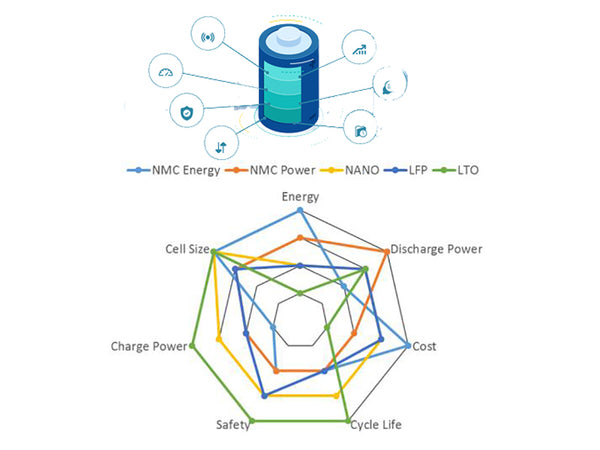The charging and discharging process of a power battery is a mutual conversion process between electrical energy and chemical energy, so no matter how the positive and negative materials of the battery change, the electrochemical principles are basically similar. The differences between different types of power batteries are mainly reflected in electrochemical properties and applicable occasions. Usually people use comparable battery parameters to analyze the characteristics of different batteries, such as battery voltage, capacity, internal resistance, energy density, power density, charge and discharge performance, cost, service life, monomer consistency and safety, etc. These parameters It is also the main index to evaluate the performance of electric vehicle power battery.

1.Several concepts about voltage and electromotive force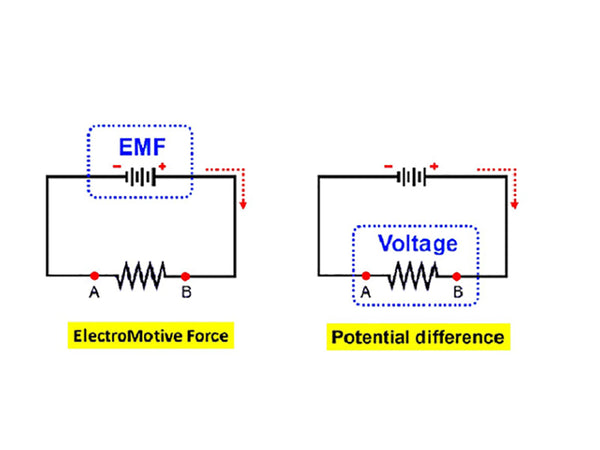The terminal voltage of the power battery refers to the potential difference between the positive and negative poles of the power battery, and the terminal voltage of the power battery when there is no load is called the open circuit voltage. The voltage in the discharged state after the power battery is connected to the load is called the load voltage, also known as the working voltage. The voltage at the end of the battery charge and discharge is called the end voltage, which is divided into the charge end voltage and the discharge end voltage. Taking the lithium manganate battery as an example, the charge-discharge curves of the battery under different states of charge (SC) are shown in Figure 1. From the discharge curve in Figure 1, it can be seen that continuing to discharge when the terminal voltage is lower than the discharge termination voltage will lead to a sharp drop in the terminal voltage and even damage the battery, so such phenomena should be strictly avoided.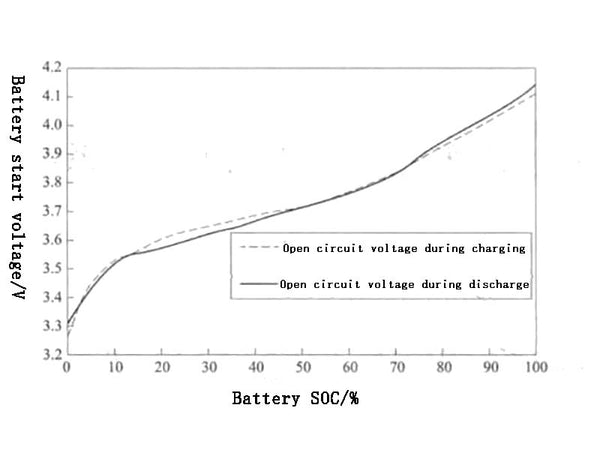Figure 1 Open circuit voltage curves of lithium manganate batteries at different states of charge (SOC)

The electromotive force of a battery is equal to the difference between the equilibrium electrode potentials of the two electrodes that make up the battery. In fact, the two electrodes in the battery are not in a thermodynamically reversible state. The electrode potential at this time is the stable electrode potential rather than the balanced electrode potential. Therefore, the open circuit voltage of the battery is theoretically not equal to the electromotive force of the battery, but generally speaking, it can be considered that The open circuit voltage of the battery is approximately equal to its electromotive force.

2.current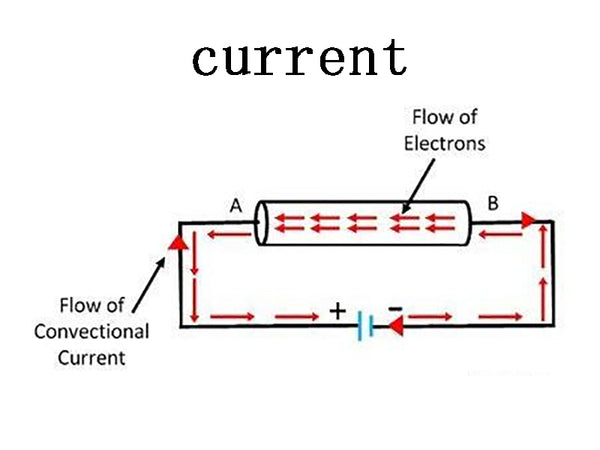The current output by the battery when discharging is called the discharging current, and the current flowing through the battery when charging is called the charging current. The maximum value of the current allowed by the battery when discharging or charging is called the maximum allowable current.

Generally speaking, the instantaneous power of the battery refers to the product of the terminal voltage of the battery and the current flowing through the electrode in a certain state. Assuming that the SOC of the battery is x at the initial moment, the open-circuit voltage of the battery can be written as Ux, the internal resistance of the battery is R0, the current flowing into (or out of) the battery controlled by the external power source is I0, the terminal voltage of the battery is U1, and the built battery is A simple model of the resistance is shown in Figure 2.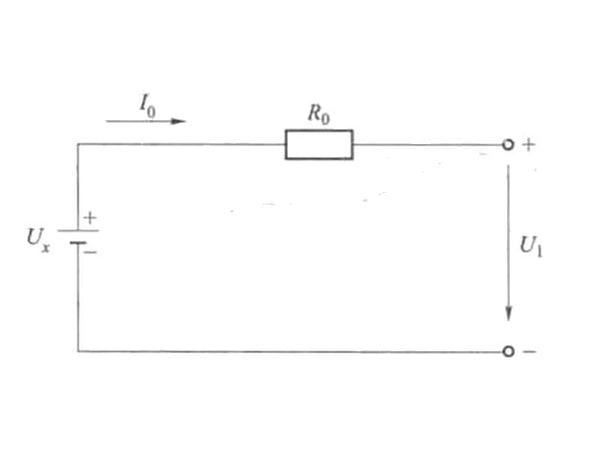Figure 2 Simple model of battery internal resistance

According to the foregoing content and the setting in Figure 2, the power of the battery when the SOC is x is:
P=U1I0=(Ux-l0R)I0

Ux in the above formula can be obtained by querying the corresponding curve between the SOC of the battery and the open circuit voltage (Figure 1). If the length of time that the battery continues to discharge with the power P is t, assuming that the SC of the battery at time t is x', the open-circuit voltage of the battery is U'x, and the internal resistance of the battery is still R0, then the current It at this time still satisfies the above formula. the equation shown, that is
P=U’1It=(U’x-ItR0)lt

The size of the charge/discharge current of the battery directly affects the various indicators of the battery. When expressing the capacity of the power battery, the charge/discharge current of the power battery must be specified, which is usually expressed by the charge/discharge rate. The charge/discharge rate is divided into time rate and rate. Two, the hour rate refers to the discharge rate expressed by the discharge time, that is, the time unit required to discharge the rated capacity with a certain discharge current is h. The rate refers to the output when the power battery discharges its rated capacity within a specified time. The current value is numerically equal to a multiple of the rated capacity. which is
I=k·Cn

In the formula: I - the charging or discharging current of the battery, A;
n—the calibrated discharge time corresponding to the rated capacity of the battery, h;
C—the rated capacity of the battery, Ah;
k is a proportional coefficient.

The meaning represented by Cn in the above formula is the amount of electricity charged or discharged for n hours, which is called the discharge rate.

3.battery capacity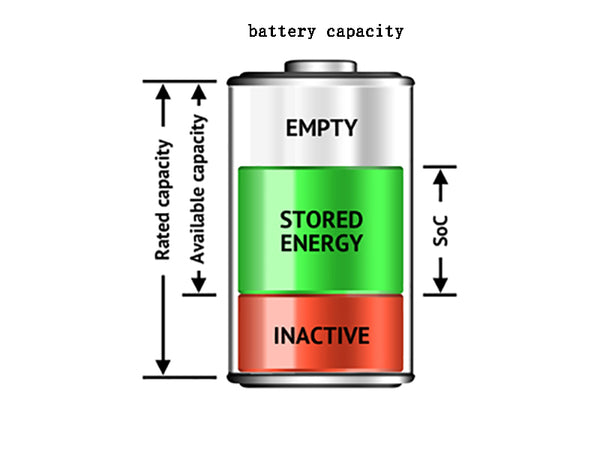The capacity of the battery refers to the power output when the fully charged battery is discharged under specified conditions until the terminal voltage reaches the termination voltage, and the unit is Ah. The capacity of the battery includes three different concepts: theoretical capacity, rated capacity and actual capacity

(1) Theoretical capacity. Assuming that all the active substances in the battery participate in the reaction, the amount of electricity that can be given according to Faraday's law is called the theoretical capacity. The theoretical capacity is the maximum limit value of the battery capacity, and the capacity released by the battery in practical applications is only a part of the theoretical capacity.

(2) Rated capacity. The rated capacity is also called the nominal capacity, which means that the rated capacity of the battery should be discharged under the specified conditions.

(3) Actual capacity. The actual capacity refers to the power that a fully charged battery can output under certain conditions, which is equal to the product of the discharge current and the discharge time.

The actual capacity of the battery is mainly affected by the discharge regime. The discharge regime includes factors such as discharge rate, discharge form (constant current, variable current or pulse), termination voltage and temperature. The termination voltage refers to the voltage at the end of charging and discharging.

4.State of charge (SOC) of the battery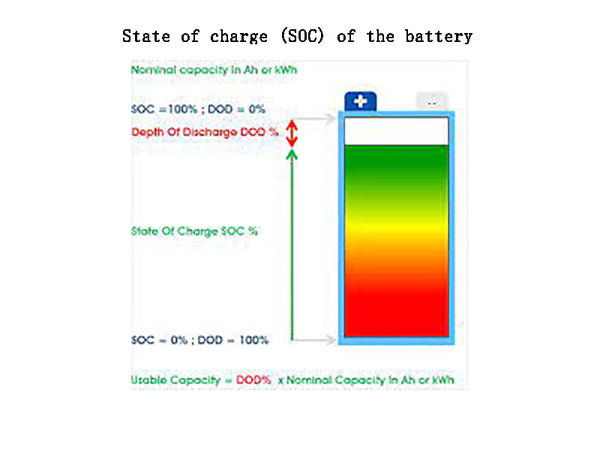The state of charge (SOC) of the battery is usually used to describe the influence mechanism of the remaining capacity of the battery to the rated capacity and the battery capacity. SOC can also be expressed as a function of battery discharge rate, operating ambient temperature, and battery aging.

5.Depth of discharge (DOD) of the battery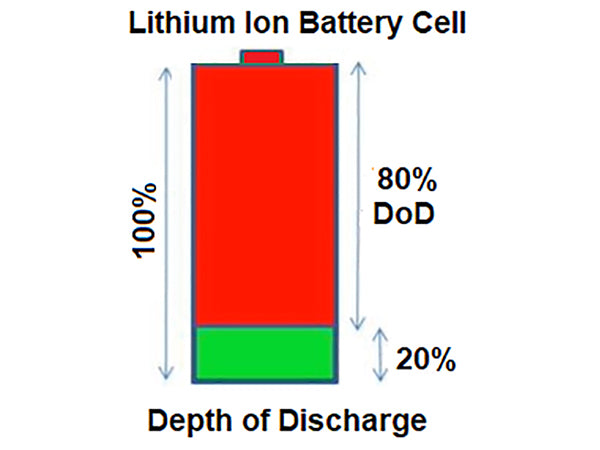Depth of discharge (DOD) is used to describe the ratio of the discharged power of the battery to the rated capacity of the battery

6.Battery energy and energy densityThe energy of the battery refers to the electrical energy output by the battery under the discharge system specified by a certain standard, and the unit is watt-hour (Wh) or kilowatt-hour (kWh).

The energy of the battery is also divided into the nominal energy and the actual energy. The nominal energy refers to the product of the battery's rated capacity and its rated voltage; the actual energy is the product of the battery's actual capacity and the average operating voltage under certain discharge conditions.

Energy density (also known as specific energy) is usually used as an important indicator to measure the performance of various power batteries. Energy density includes mass energy density and volume energy density. Mass energy density refers to the electrical energy that the battery can output per unit mass, in watt-hours per kilogram (Wh/kg); volumetric energy density refers to the electrical energy that the battery can output per unit volume, in watt-hours per liter (WhL). Since the battery's available capacity is a function of the battery's discharge rate, the definitions of the battery's mass energy density and volumetric energy density are also related to the battery's discharge rate.

7.Battery power and power density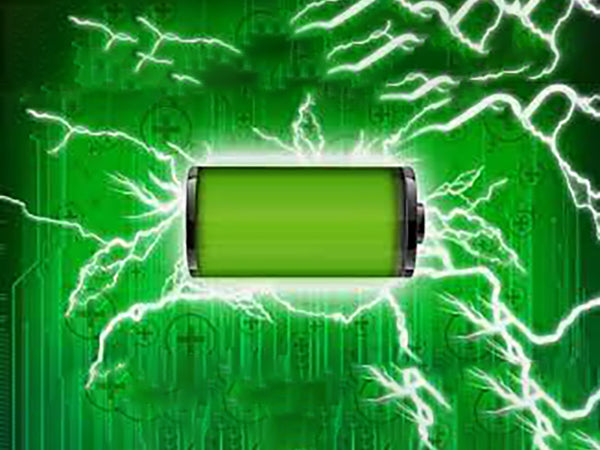The power of the battery refers to the energy output by the battery in a unit of time under a certain discharge regime, in watts (W) or kilowatts (kW). To test the peak power of the battery, a variety of approximate calculation methods have been proposed, and these methods are also used to evaluate the power characteristics of the battery. The American FreedomCAR project proposes a hybrid pulse power characteristic (HPPC) test method. The purpose of this test method is to verify whether the peak charge and discharge power of the battery meets the goals proposed by Freedom CAR.

The HPPC test procedure is shown in Table 1, and the corresponding pulse current curve is shown in Figure 3. In Figure 3, t0-t4 are the sampling points of the test data. The test uses the maximum discharge current Imax provided by the battery manufacturer as the reference discharge current, the pulse charging current is 0.75Imax, and the durations of the charging pulse current and the discharging pulse current are each 10s. The interval between pulses is 40s, and by calculating ΔV/ΔI, the discharge impedance Rdch and the charge impedance Rch are obtained 10s after the start of the pulse. The current direction is positive when the battery is discharging, and the current direction is negative when the battery is charging.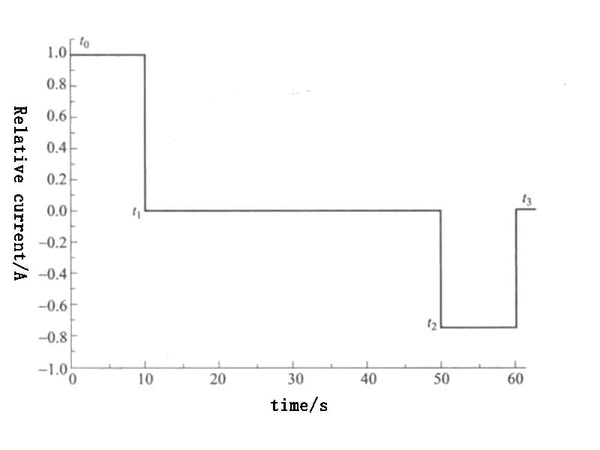Figure 3 HPPC pulse current curve

The discharge impedance Rdch and charging impedance Rch in the test are respectively
Rdch=△Udch/△Idch=(Ut1-Ut0)/-(It1-It0)=(Ut1-Ut0)/(It0-It1)
Rch=△Uch/△Ich=(Ut3-Ut2)/-(It3-It2)=(Ut3-Ut2)/(It2-It3)

Substitute the charging impedance and discharging impedance calculated by the above two formulas into the following two formulas to obtain the pulse discharge power Pdch and pulse charging power Pch of the battery.
Pdch=Umin·(Uocv-Umin)/Rdch

In the formula: Umin—the minimum voltage that the battery allows to discharge, V;
Uoxv—the open circuit voltage of the battery, corresponding to the depth of discharge (DOD) of the battery, which can be obtained by querying the SOC—OCV curve, V.
Pch=Umax·(Umax-Uocv)/Rch

In the formula: Umax—the highest voltage of battery charging, V.

In order to measure the pulse discharge power and pulse charge power of the battery under different DODs, the HPPC power test method shown in Figure 4 can be used.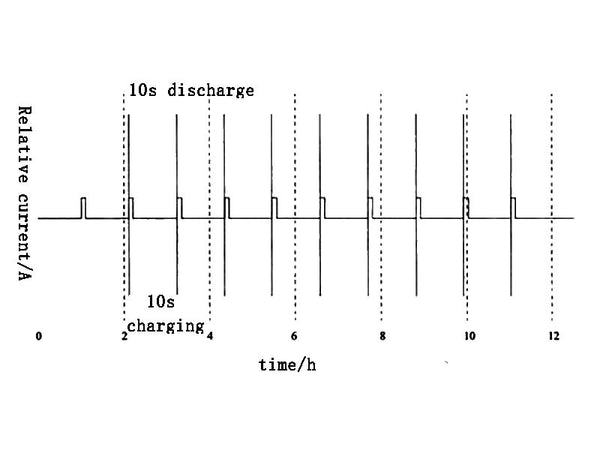Figure 4 HPPC power test method

① Discharge the fully charged battery with a current of 1C, and adjust the DOD of the battery to the target value;

②After standing for 1h, it will return to the electrochemical and thermal equilibrium state

③ Perform a pulse charge with a duration of 10s and a pulse discharge with a duration of 10s on the battery;

④Repeat the test contents of ①~③, and carry out a test every 10% DXOD. Finally, the relationship between the pulse charge and discharge power of the battery and DOD is obtained as shown in Figure 5.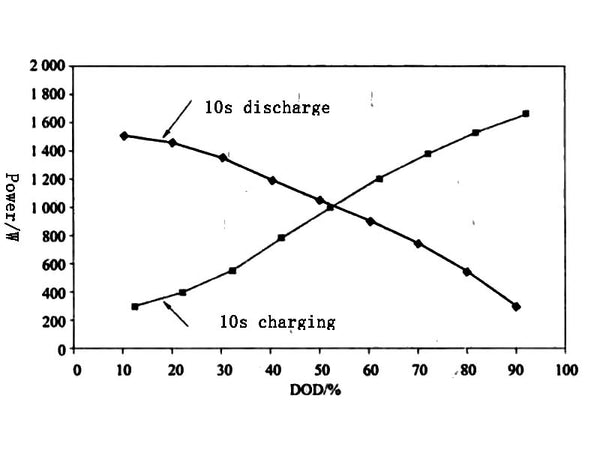Figure 5. The relationship between charge/discharge power and DOD

8.Mass Power Density and Volume Power Density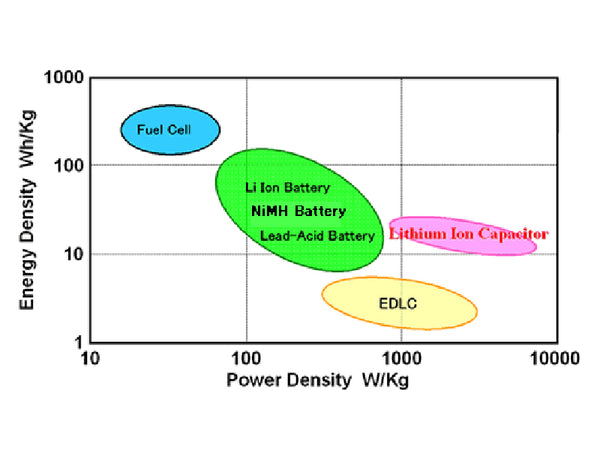Mass power density refers to the power that a unit mass of battery can output, in W/kg. Volume power density refers to the power that a unit volume of battery can output, and the unit is W/L. Mass power density is an important indicator to evaluate whether the battery can meet the acceleration and climbing performance of electric vehicles. For electrochemical cells, the mass power density (specific power) is closely related to the depth of discharge (D) of the cell. Therefore, the depth of discharge (DOD) of the battery is also indicated when expressing the mass power density of the battery.

9.The cycle life of the battery (cyele life)The cycle life of the battery refers to the charging and discharging of the battery before the battery capacity drops to a specified value (generally specified as 80% of the rated value) according to a certain test standard. The total number of cycles. Cycle life is an important indicator for evaluating battery life performance.

Figure 6 shows the voltage curves of the battery charged/discharged at a rate of 1C under different cycle times. Before the cycle test, the battery needs to be charged and discharged at a rate of 0.5C for 50 cycles to establish a stable SEI. membrane. From the analysis of the deterioration of the charge-discharge curve, the possible reasons for the deterioration of the battery include the deterioration of the positive electrode active material or the loss of lithium ions that can be extracted.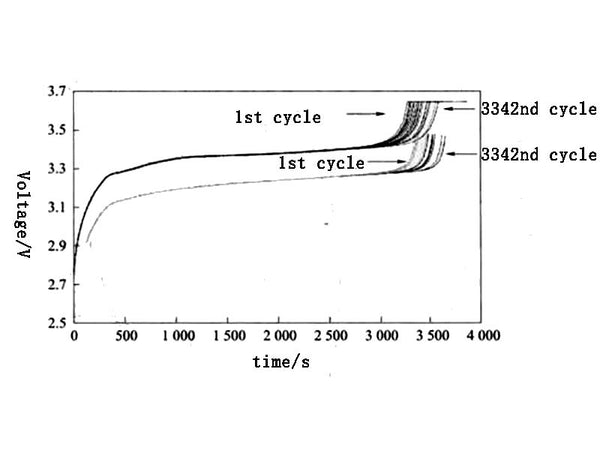Figure 6. Charge-discharge voltage curves at 1C rate for different cycles

Figure 7 shows the changes in capacity, ampere-hour efficiency and watt-hour efficiency of the battery under different cycle times. It can be seen from Figure 7 that after 3500 cycles, the remaining capacity of the battery is about 90% of the initial value, indicating that the battery system has high stability and small side reactions at room temperature.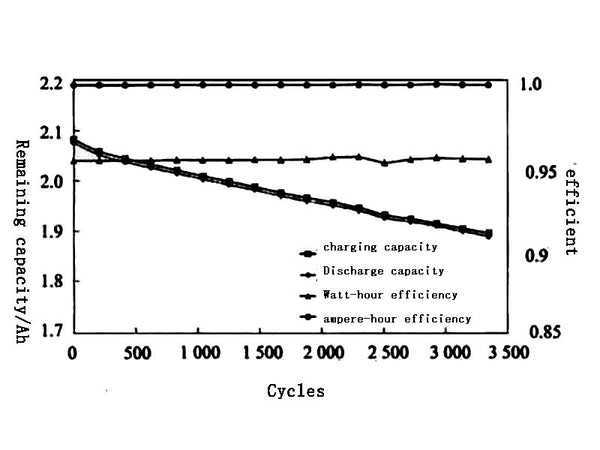Figure 7 Changes in capacity, ampere-hour efficiency and watt-hour efficiency of the battery under different cycles

10.Self-discharge rate of the battery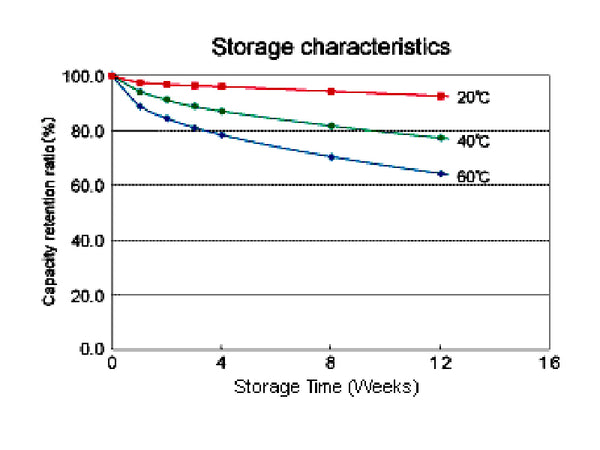The self-discharge rate of a battery refers to the rate of decrease in the capacity of the battery during storage, that is, the rate at which the battery loses capacity due to self-discharge when there is no load. The self-discharge rate C is expressed by the percentage of capacity reduction per unit time, that is
C=[(Cini-Ctin)/Cini·100%]/t=(Cini-Ctin)/(Cini·t)·100%

In the formula: Cini—the capacity of the battery at the initial moment of storage, A h;
Ctin - the capacity of the battery after storage, A h;
t—the battery storage time, days/months.

The self-discharge rate is usually related to time and temperature. The higher the ambient temperature, the more obvious the self-discharge phenomenon is. Therefore, the battery should regularly check its state of charge during long-term storage, and store it under suitable temperature and humidity conditions.

11.The output efficiency of the battery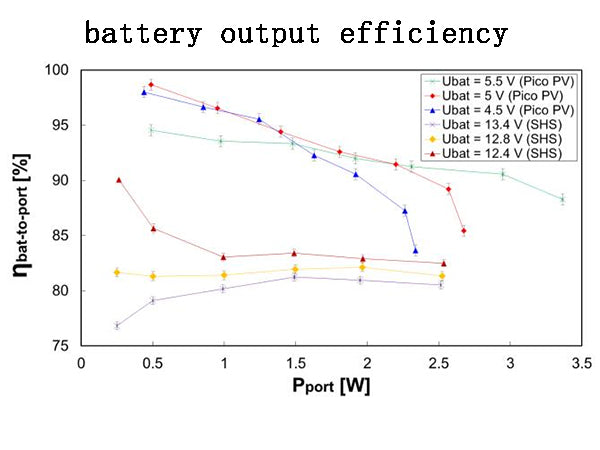The battery is actually an energy storage. When charging, it converts electrical energy into chemical energy and stores it, and when discharging, it converts chemical energy into electrical energy and releases it for use by electrical devices. The output efficiency of a battery is usually expressed in terms of capacity efficiency and energy efficiency. The capacity efficiency of a battery refers to the ratio of the output capacity of the battery when it is discharged to the input capacity when it is charged, also known as the ampere-hour efficiency. The energy efficiency of a battery refers to the ratio of the energy output when the battery is discharged to the energy input when charging, also known as the watt-hour efficiency. As shown in Figure 7, the ampere-hour efficiency of the A123 lithium iron phosphate battery is relatively high (close to 100%), and the watt-hour efficiency is also around 95%, indicating that the battery has a high output efficiency. For electric vehicles, energy efficiency is a more important evaluation index than capacity efficiency.

12.Battery ConsistencyThe existence of parameters such as voltage, internal resistance, and capacity between batteries of the same type, specification, and model is also called battery consistency. The life of a set of batteries is largely determined by their consistency. Since the power batteries of electric vehicles are used in groups, consistency is one of the key indicators for evaluating the performance of battery packs. The factors affecting the battery consistency mainly include the design and manufacturing level of the single cell, the temperature field distribution of the battery pack, etc.

13. Anti-abuse ability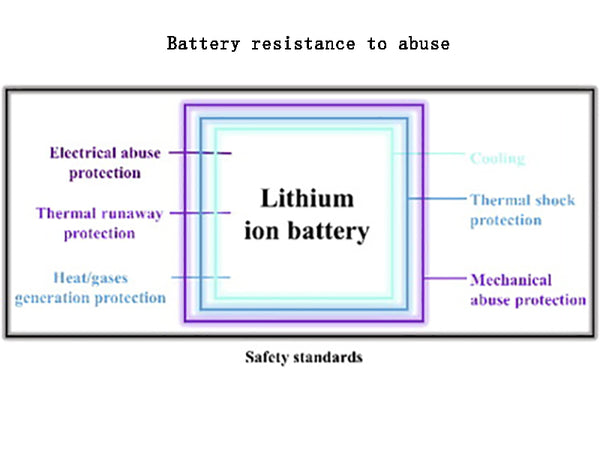The battery's anti-abuse ability refers to the battery's resistance to short-circuit, overcharge, over-discharge, mechanical vibration, impact, extrusion, and abnormal use conditions such as high temperature and fire.

14.The structure of the power battery(1) Single power battery: the smallest unit that constitutes a battery, generally composed of a positive electrode, a negative electrode and an electrolyte.

(2) Power battery module: A single power battery is a combination of modules formed by series and parallel.

(3) Power battery pack: It consists of one or more power battery modules.

(4) Battery management system: a system that can control the input and output power of the power battery, monitor the status of the battery (temperature, voltage, state of charge), and provide a communication interface for the battery.

(5) Battery auxiliary devices: battery brackets, thermal management systems and other components required for the normal operation of the battery system.

(6) Power battery system: the combination of all power battery packs, battery management systems and battery auxiliary devices.

15.Discharge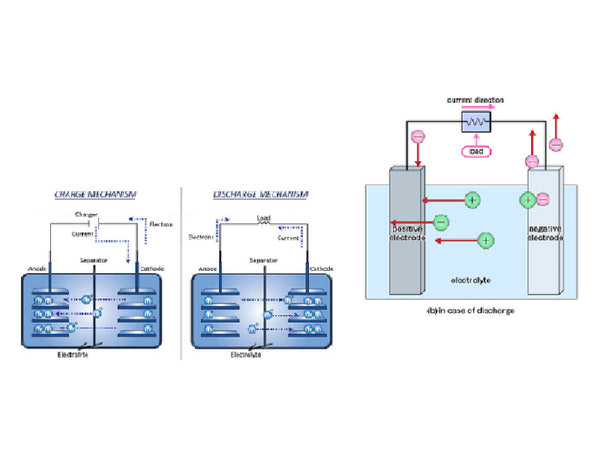(1) Constant current discharge: The power battery is discharged with a controlled constant current.

(2) Rate discharge: the discharge of the power battery at the rate value of the rated current.

16.Charge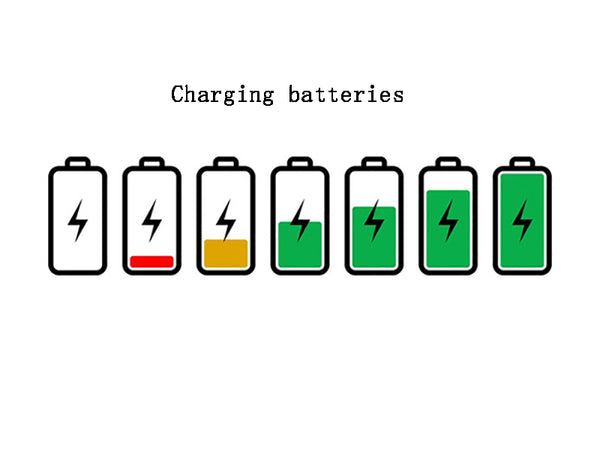(1) Trickle charging: In order to supplement self-discharge and keep the power battery in a state of approximately full charge, it is only suitable for some batteries.

2) Balanced charging: a charging method adopted by the battery management system to ensure the uniform state of charge of all single power batteries in the power battery pack.

(3) Constant current charging: a method of charging the power battery with a controlled constant current

(4) Pulse charging: the method of charging the power battery with pulse current

17.Related phenomena(1) Internal short circuit: The phenomenon of micro-short circuit or short circuit between the positive and negative electrodes inside the power battery.

(2) Gas evolution: the phenomenon that the power battery generates gas during the charging process.

(3) Thermal runaway: During the charging and discharging process of the battery, the power battery is damaged due to a cumulative and mutually reinforcing effect caused by the current and temperature inside the battery.

(4) Reverse polarity: the phenomenon that the normal polarity of the power battery changes.

(5) Leakage: the phenomenon that the electrolyte leaks to the outside of the power battery.

(6) Memory effect: After a long-term shallow charge/discharge cycle, the power battery exhibits obvious capacity loss and a drop in discharge voltage when it is deeply discharged.

(7) Overcharging: the phenomenon that the power battery continues to charge after it has reached a fully charged state, and overcharging can easily lead to gas evolution and thermal runaway.

(8) Overdischarge: the phenomenon that the terminal voltage of the power battery continues to discharge when it is lower than the discharge termination voltage, and the overdischarge can easily cause damage to the electrode active material.